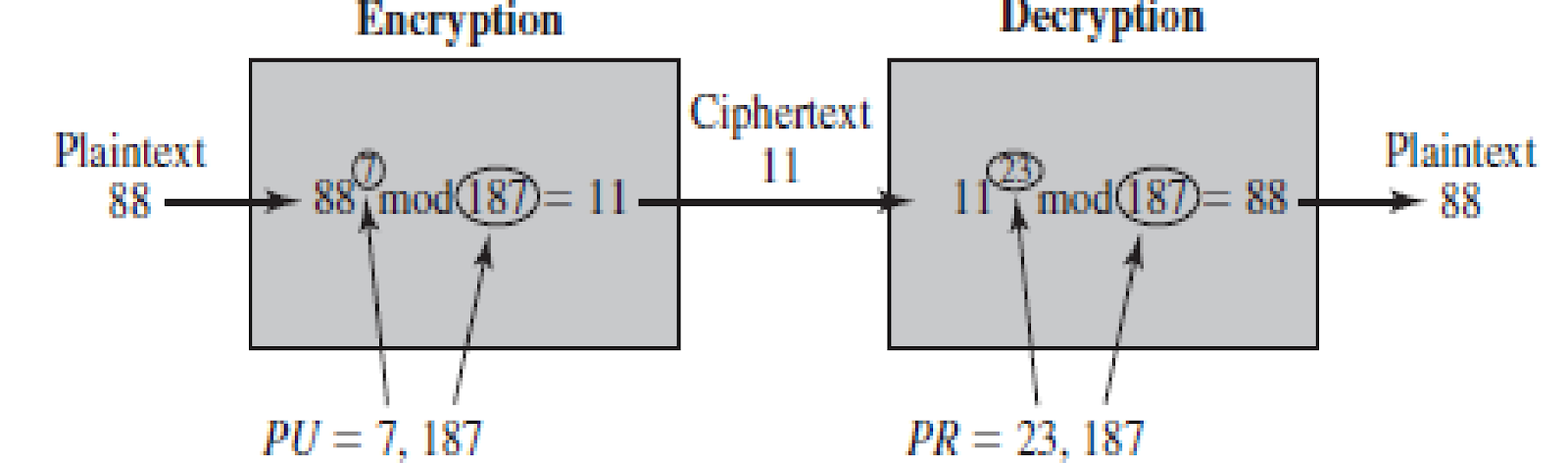# Explain RSA algorithm with example ?

RSA (Rivest–Shamir–Adleman) is an algorithm used by modern computers to encrypt and decrypt messages. It is an asymmetric cryptographic algorithm. Asymmetric means that there are two different keys. This is also called public key cryptography, because one of the keys can be given to anyon

• The concept of public key cryptography was introduced by Diffie and Hellman in 1976; many algorithms proposed:
• RSA - Developed in 1977 at MIT by Ron Rivest, Adi Shamir & Len Adleman
• Most widely used general-purpose approach to public-key encryption
•  The RSA  scheme is a cipher in which the plaintext and ciphertext are integers between 0 and n -  1 for some n . A typical size for n  is 1024 bits, or 309 decimaldigits. That is, n  is less than 21024 .

Algorithm Requirements

•  For this algorithm to be satisfactory for public-key encryption, the following requirements must be met:
1.  It is possible  to find values of e, d, n   such that Med mod n = M for all M < n
2.  It is computationally easy to calculate C=Me  mod n and Cd mod n for all values of M < n
3.  It is infeasible (computationally difficult)  to determine d given e  and n

The RSA algorithm

Key generation by Alice(receiver)

• Select two large prime numbers p, q
• Calculate n=p x q
• Calculate ø(n) =  (p-1) x (q-1)               (refer back for Euler’s phi function ø(n)
• Select e which is < ø(n) and relatively prime to ø(n)
• Calculate d= e -1 mod ø(n)  ( d is the multiplicative inverse of e in mod ø(n) )
• Then Public key (of the receiver) is PU= {e,n} and private key is  PR= {d, n}

Encryption by Bob with Alice’s public key:

Let the plaintext is encoded into an integer value M, such that M< n. Then ciphertext C=Me mod n

Decryption by Alice with Alice’s private key:

The ciphertext C is received. Then plaintext M = Cd mod n

Example of RSA Algorithm

1. Select two prime numbers, p = 17 and q = 11.

2. Calculate n = pq = 17 * 11 = 187.

3. Calculate ø(n) = (p - 1)(q - 1) = 16 * 10 = 160.

4. Select e such that e is relatively prime to ø(n) = 160 and less than ø(n) ; we choose e = 7.

5. Determine d such that de = 1 (mod 160) and d < 160. The correct value is d = 23, because 23 * 7 = 161 = (1 * 160) + 1; d can be calculated using the Extended Euclidean algorithm